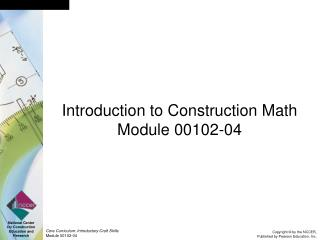# Introduction to Construction Math Module 00102-04 - PowerPoint PPT PresentationDownload PresentationIntroduction to Construction Math Module 00102-04

Introduction to Construction Math Module 00102-04Download Presentation## Introduction to Construction Math Module 00102-04

- - - - - - - - - - - - - - - - - - - - - - - - - - - E N D - - - - - - - - - - - - - - - - - - - - - - - - - - -
##### Presentation Transcript

1. Introduction to Construction Math Module 00102-04

2. Transparency 1 Objectives Upon completion of this module, you will be able to do the following: 1. Add, subtract, multiply, and divide whole numbers, with and without and without a calculator.   2. Use a standard ruler and a metric ruler to measure.   3. Add, subtract, multiply, and divide fractions.   4. Add, subtract, multiply, and divide decimals, with and without a calculator.   5. Convert decimals to percentages and percentages to decimals.   6. Convert fractions to decimals and decimals to fractions. 7. Explain what the metric system is and how it is important in the construction trade. 8. Recognize and use metric units of length, weight, volume, and temperature. 9. Recognize some of the basic shapes used in the construction industry, and apply basic geometry to measure them.

3. Transparency 2 Figure 3 - Counting out the wrenches

4. Transparency 3 Figure 6 - Types of measurement tools

5. Transparency 4 Figure 8 - The architect’s scale

6. Transparency 5 Figure 10 - Identify the measurement

7. Transparency 6 Figure 14 - Showing 1.3 inches on a machinist’s rule

8. Transparency 7 Figure 19 - 100-Gallon-capacity tank

9. Transparency 8 Figure 20 - What type of measurement is it?

10. Transparency 9 Figure 22 - Metric ruler

11. Transparency 10 Figure 29 - Typical angles

12. Transparency 11 Figure 30 - Common shapes

13. Transparency 12 Figure 32 - Cutting a rectangle on the diagonal produces two right triangles

14. Transparency 13 Figure 33 - Cutting a square on the diagonal produces two right triangles

15. Transparency 14 Figure 34 - The sum of a triangle’s three angles always equals 180 degrees

16. Transparency 15 Figure S-2 - Types of triangles

17. Transparency 16 Figure 35 - Measurements that apply to circles

18. Transparency 17 Figure 36 - Volume of a rectangle

19. Transparency 18 Figure 37 - Volume of a cube

20. Transparency 19 Figure 38 - Volume of a cylinder

21. Supplemental Art

22. Figure 1 - Place values

23. Figure 2 - Carrying in addition

24. Figure 4 - Parts of a calculator

25. Figure 5 - From decimal remainder to whole number remainder

26. Figure 7 - Distances on the standard ruler

27. Figure 9 - Review questions ruler

28. Figure 11 - 3/4 of a pizza

29. Figure 12 - 5/8 of a pizza

30. Figure 15 - Study problems machinist’s rule

31. Figure 16 - Review questions machinist’s rule

32. Figure 17 - Pipe coating

33. Figure 18 - Fuel tank

34. Figure 21 - The decimal scale

35. Figure 23 - Study problems metric ruler

36. Figure 24 - 72 inches of material

37. Figure 25 - 72 centimeters of material

38. Figure 26 - Review questions 1-4

39. Figure 27 - Review questions 5-7

40. Figure 28 - Review questions 8 and 9

41. Figure 31 - What common shapes can you find at this construction site?

42. Measurement Memory Tools

43. 3/4/5 Rule

44. Calculating the Area of a Cylinder